# Civil Engineering - Highway Engineering

### Exercise :: Highway Engineering - Section 4

41.

If V is the design speed of vehicles in km/hour, the change of radial acceleration in metres/sec3, is

 A.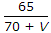B.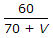C.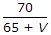D.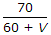E. 80/(75+V)

Explanation:

No answer description available for this question. Let us discuss.

42.

Volume of traffic which is due to improvement carried out in adjacent area, is known as

 A. development traffic B. generated traffic growth C. normal traffic growth D. current traffic.

Explanation:

No answer description available for this question. Let us discuss.

43.

Roughness index of roads, is expressed as

 A. size of the stone on the pavement B. number of patches on the pavement C. cumulative deformation of surface per horizontal distance D. type of the road surface.

Explanation:

No answer description available for this question. Let us discuss.

44.

If N is deviation angle, the length L, of a parabolic vertical curve for safe stopping distance S, is

 A.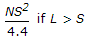B.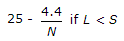C.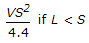D.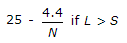E. both (a) and (b)

Explanation:

No answer description available for this question. Let us discuss.

45.

Pick up the correct statement from the following:

 A. The height of head light above road surface, is taken as 0.75 m B. The beam of head light is up to one degree upwards from the grade of road C. While designing the valley curve, the height of the object is assumed nil D. All the above